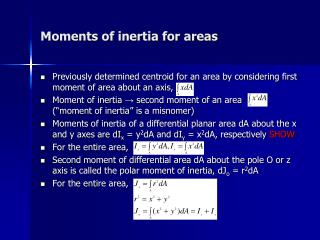DownloadDownload PresentationMoments of inertia for areas

# Moments of inertia for areas

Download Presentation## Moments of inertia for areas

- - - - - - - - - - - - - - - - - - - - - - - - - - - E N D - - - - - - - - - - - - - - - - - - - - - - - - - - -
##### Presentation Transcript

1. Moments of inertia for areas • Previously determined centroid for an area by considering first moment of area about an axis, • Moment of inertia → second moment of an area (“moment of inertia” is a misnomer) • Moments of inertia of a differential planar area dA about the x and y axes are dIx = y2dA and dIy = x2dA, respectively SHOW • For the entire area, • Second moment of differential area dA about the pole O or z axis is called the polar moment of inertia, dJo = r2dA • For the entire area,

2. Parallel-Axis Theorem • SHOW • However, x’ passes through the centroid of the area • Similarly,

3. Radius of Gyration of an Area • A quantity often used in the design of columns • (units are length)

4. Moments of inertia for an area by integration (Case 1) • Specify the differential element dA (usually a rectangle with finite length and differential width) • Differential element located so it intersects the boundary of the area at an arbitrary point (x,y) • Case 1: • Length of element is oriented parallel to axis, SHOW • Iy determined by a direct application of since element has an infinitesimal thickness dx (all parts of the element lie at the same moment-arm distance x from the y-axis)

5. Moments of inertia for an area by integration (Case 2) • Case 2: • Length of element is oriented perpendicular to axis, SHOW • does not apply, all parts of the element will not lie at the same moment-arm distance from axis • First calculate moment of inertia of the element about a horizontal axis passing through the element’s centroid • Determine moment of inertia of the element about the x-axis by using parallel-axis theorem • Integrate to yield Ix • EXAMPLES (pg 523)

6. Moments of inertia for composite areas • Divide the area into its “simpler” composite parts • Determine the perpendicular distance from the centroid of each part to the reference axis (often centroid of entire area) • Determine the moment of inertia of each part about its centroidal axis (parallel to the reference axis) • If the centroidal axis does not coincide with the reference axis, apply the parallel-axis theorem to determine the moment of inertia of the part about the reference axis • The moment of inertia of the entire area about the reference axis is obtained by summing the results of its parts (“holes” are subtracted) • EXAMPLES (pg 530)